# Apache Beam

## What is Dataflow

Dataflow is a software paradigm based on the idea of disconnecting computational actors into stages (pipelines) that can execute concurrently.
-The All-Knowing Wiki

## Executed Concurrently

### Huh?

Google took their Cloud Dataflow product, and donated the API, local runner, and some other integrations to the Apache project. While Beam is still incubating, it is receiving updates as the public APIs require it and is being worked on by non-Googlers, in addition to the Google team. The hope is to avoid vendor lock in and provide more flexibility in terms of running environment.

## Executed Concurrently

###Google Cloud DataFlow

Apache Beam is the API. Google Cloud Dataflow is a "Runner"

Other runners supporting by Apache Beam:

• Apache Spark
• Direct (Local)

## Disconnecting ActorsSource - Where data comes from.

• Stream
• Files
• Database

Transform - An execution stage. It literally transforms the input data stream into some new datastream. These may be comprised of other and multiple transforms.

Sink - Where data goes to.

• Stream
• Files
• Database

## Example

I'll use the Dataflow SDK examples.

These are Java programs. The Python API is experimental.

## Example: Term Frequency -- Inverse Document Frequency

TF-IDF is a common and basic measure used to identify how important a word is in a document. It is often a first step when building a textual search engine.

$$\mathrm {tfidf} (t,d,D)=\mathrm {tf} (t,d)\cdot \mathrm {idf} (t,D)$$

$$\mathrm {tf} (t,d) = |\{i \in d\ : i = t\}|$$

$$\mathrm {idf} (t,D) = \log \frac{|D|}{|\{d \in D: t \in d\}|}$$

## Example: TF-IDF Steps


pipeline
// Compute Everything
.apply(new ComputeTfIdf())
.apply(new WriteTfIdf(options.getOutput()));

pipeline.run();


## First notes

The ComputeTfIdf function is now forcibly separated from the IO.

Must consider how to break problem apart ahead of time. Once a step is done, there is no going back.

## Example: TF-IDF Steps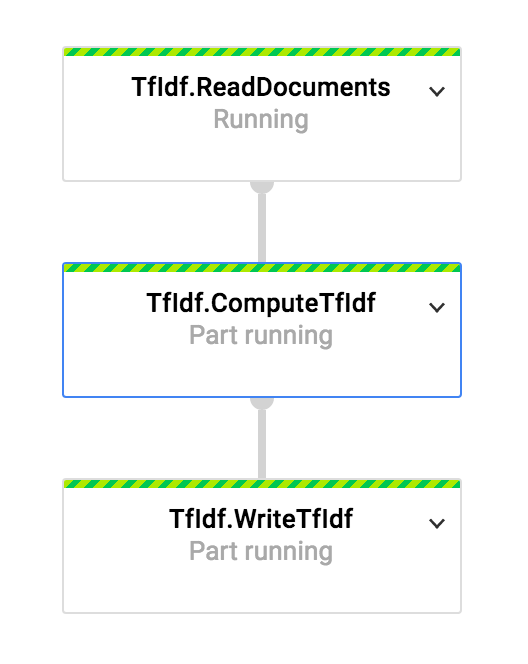## Example: TF-IDF Steps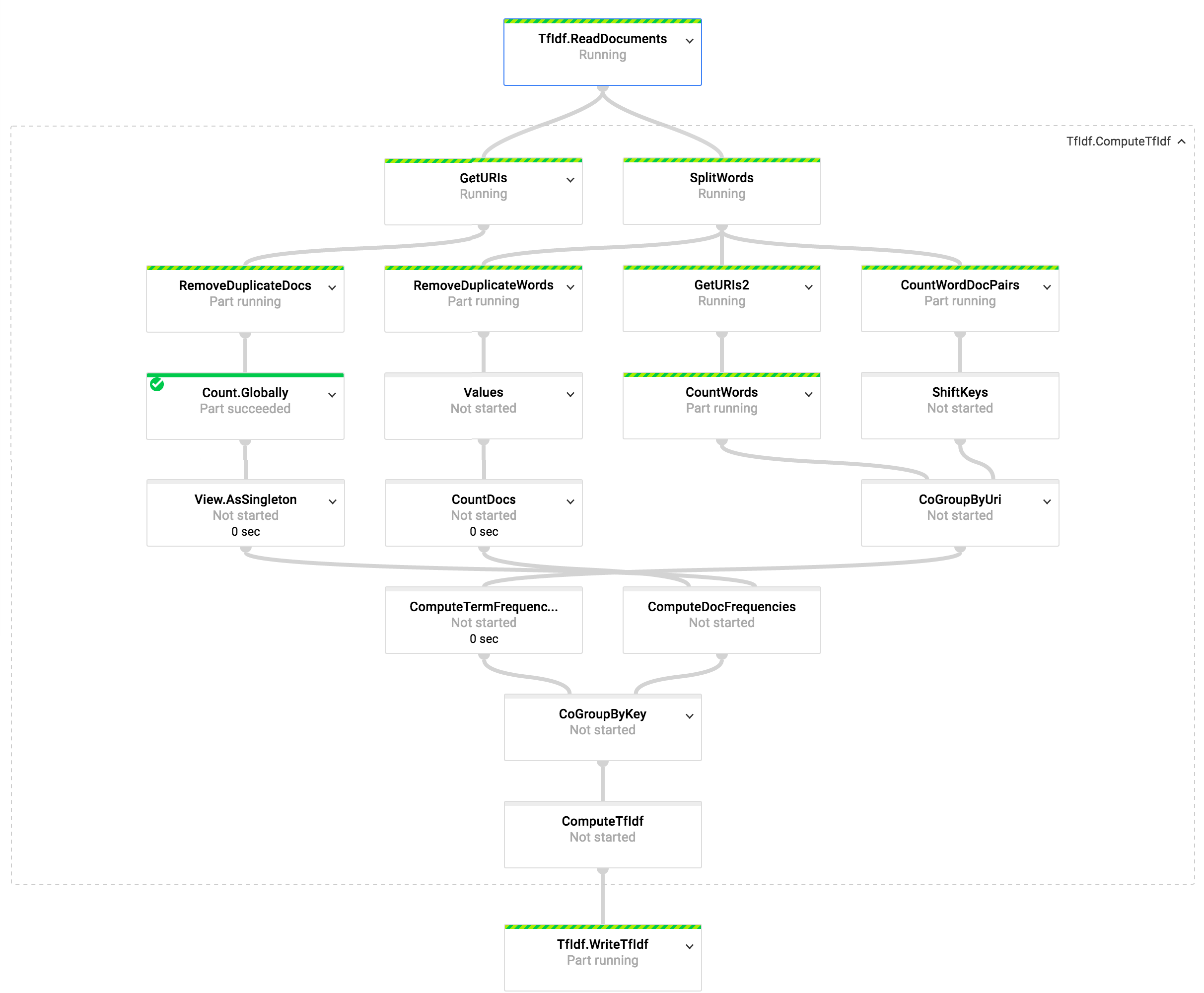## Example: TF-IDF Steps

$$\mathrm {tfidf} (t,d,D)=\mathrm {tf} (t,d)\cdot \mathrm {idf} (t,D)$$

$$\mathrm {tf} (t,d) = |\{i \in d\ : i = t\}|$$

$$\mathrm {idf} (t,D) = \log \frac{|D|}{|\{d \in D: t \in d\}|}$$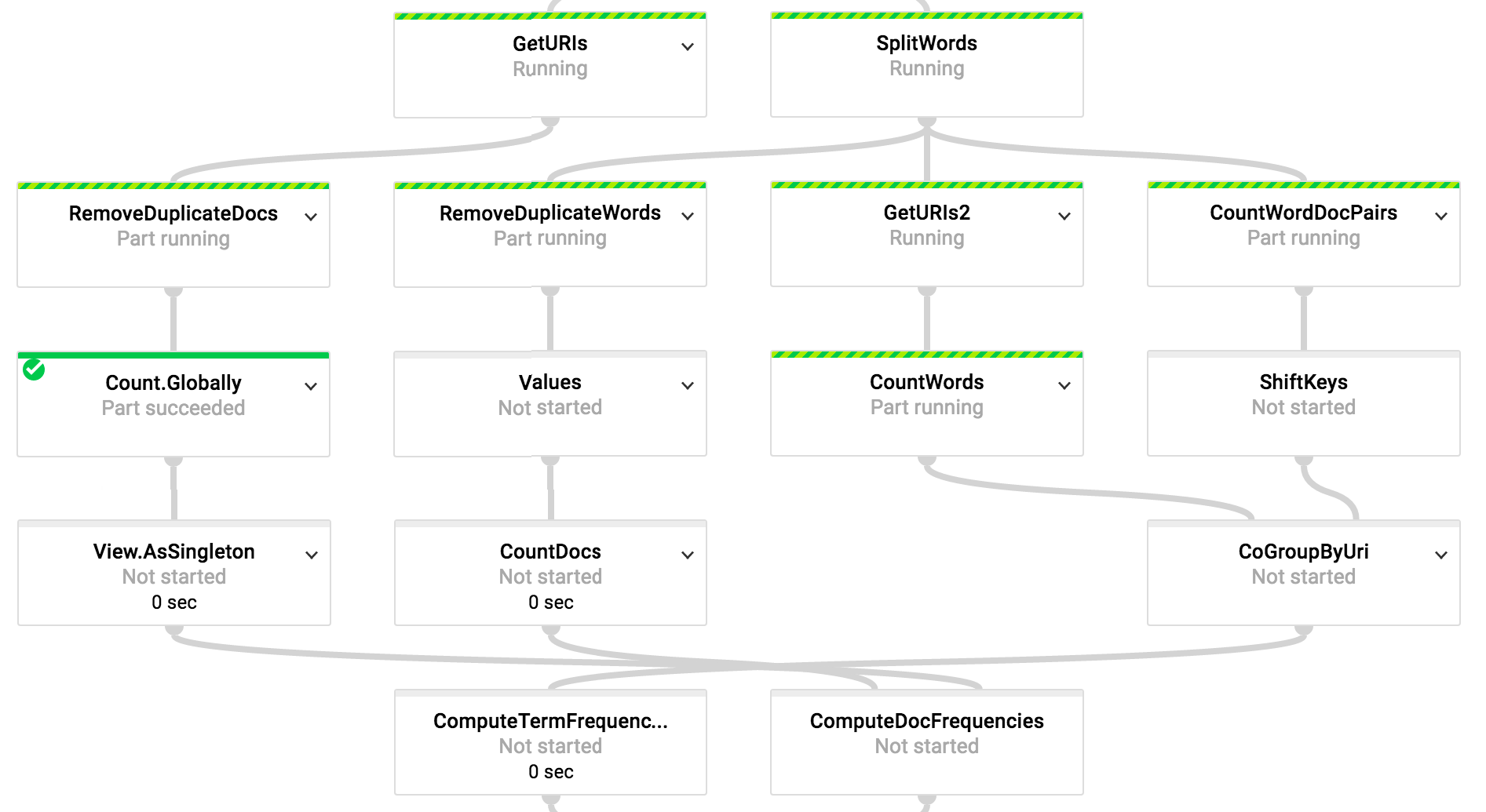## Document Count

// Compute the total number of documents, and
// prepare this singleton PCollectionView for
// use as a side input.
final PCollectionView<Long> totalDocuments =
uriToContent
.apply("GetURIs", Keys.<URI>create())
.apply("RemoveDuplicateDocs", RemoveDuplicates.<URI>create())
.apply(Count.<URI>globally())
.apply(View.<Long>asSingleton());


## Splitting Words

PCollection<KV<URI, String>> uriToWords = uriToContent
.apply(ParDo.named("SplitWords").of(
new DoFn<KV<URI, String>, KV<URI, String>>() {
@Override public void processElement(ProcessContext c) {
URI uri = c.element().getKey();
String line = c.element().getValue();
for (String word : line.split("\\W+")) {
if (word.toLowerCase().equals("love"))
LOG.info("Found {}", word.toLowerCase());
if (!word.isEmpty())
c.output(KV.of(uri, word.toLowerCase()));
}
}
}));


## Computing other statistics

// Compute a mapping from each word to the total
// number of documents in which it appears.
PCollection<KV<String, Long>> wordToDocCount = uriToWords
.apply("RemoveDuplicateWords", RemoveDuplicates.<KV<URI, String>>create())
.apply(Values.<String>create())
.apply("CountDocs", Count.<String>perElement());

// Mapping from each URI to the number of words in the document
PCollection<KV<URI, Long>> uriToWordTotal = uriToWords
.apply("GetURIs2", Keys.<URI>create())
.apply("CountWords", Count.<URI>perElement());

// Count, for each (URI, word) pair, the number of
// occurrences of that word in the document associated with the URI.
PCollection<KV<KV<URI, String>, Long>> uriAndWordToCount = uriToWords
.apply("CountWordDocPairs", Count.<KV<URI, String>>perElement());


## Joining Streams by Key


// Prepare to join the mapping of URI to (word, count) pairs with
// the mapping of URI to total word counts, by associating
// each of the input PCollection<KV<URI, ...>> with
// a tuple tag. Each input must have the same key type, URI
// in this case. The type parameter of the tuple tag matches
// the types of the values for each collection.
final TupleTag<Long> wordTotalsTag = new TupleTag<Long>();
final TupleTag<KV<String, Long>> wordCountsTag =
new TupleTag<KV<String, Long>>();
KeyedPCollectionTuple<URI> coGbkInput = KeyedPCollectionTuple
.of(wordTotalsTag, uriToWordTotal)
.and(wordCountsTag, uriToWordAndCount);


## Compuing Term Frequency

// Compute a mapping from each word to a (URI, term frequency) pair
PCollection<KV<String, KV<URI, Double>>> wordToUriAndTf = uriToWordAndCountAndTotal
.apply(ParDo.named("ComputeTermFrequencies").of(
new DoFn<KV<URI, CoGbkResult>, KV<String, KV<URI, Double>>>() {
@Override public void processElement(ProcessContext c) {
URI uri = c.element().getKey();
Long wordTotal = c.element().getValue().getOnly(wordTotalsTag);
for (KV<String, Long> wordAndCount
: c.element().getValue().getAll(wordCountsTag)) {
String word = wordAndCount.getKey();
Long wordCount = wordAndCount.getValue();
Double wordCountTemp = wordCount.doubleValue();
Double wordTotalTemp = wordTotal.doubleValue();
Double termFrequency = wordCount / wordTotal;
c.output(KV.of(word, KV.of(uri, termFrequency)));
}
}
}));


## Compuing Document Frequency


// Compute a mapping from each word to its document frequency.
PCollection<KV<String, Double>> wordToDf = wordToDocCount
.apply(ParDo
.named("ComputeDocFrequencies")
.withSideInputs(totalDocuments)
.of(new DoFn<KV<String, Long>, KV<String, Double>>() {
@Override
public void processElement(ProcessContext c) {
String word = c.element().getKey();
Long documentCount = c.element().getValue();
Long documentTotal = c.sideInput(totalDocuments);
Double documentFrequency = documentCount.doubleValue()
/ documentTotal.doubleValue();

c.output(KV.of(word, documentFrequency));
}
}));


## Compuing Document Frequency

// Compute a mapping from each word to a (URI, TF-IDF) score for each URI.
PCollection>> wordToUriAndTfIdf = wordToUriAndTfAndDf
.apply(ParDo.named("ComputeTfIdf").of(
new DoFn, KV>>() {
@Override
public void processElement(ProcessContext c) {
String word = c.element().getKey();
Double df = c.element().getValue().getOnly(dfTag);

for (KV uriAndTf : c.element().getValue().getAll(tfTag)) {
URI uri = uriAndTf.getKey();
Double tf = uriAndTf.getValue();
Double tfIdf = tf * Math.log(1 / df);
c.output(KV.of(word, KV.of(uri, tfIdf)));
}
}
}));


## I hope this makes more sense now## Running the Example


mvn exec:java
-Dexec.args="--runner=DataflowPipelineRunner --project=YOUR-PROJECT
--stagingLocation=gs://YOUR-STAGING --output=gs://YOUR-OUTPUT"


## Sessions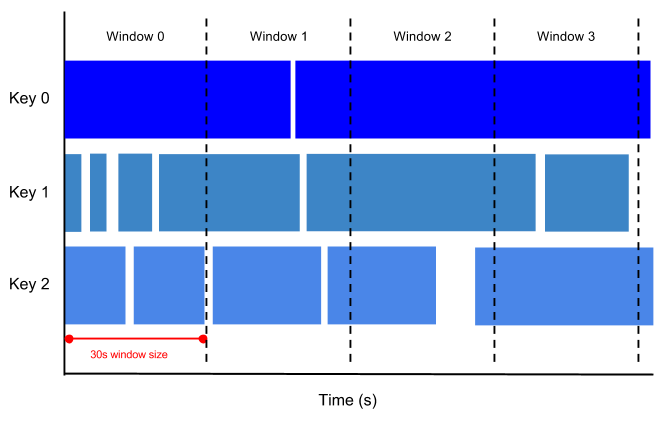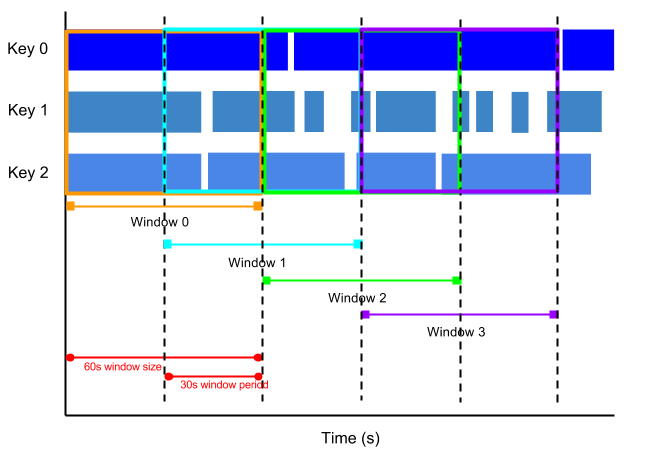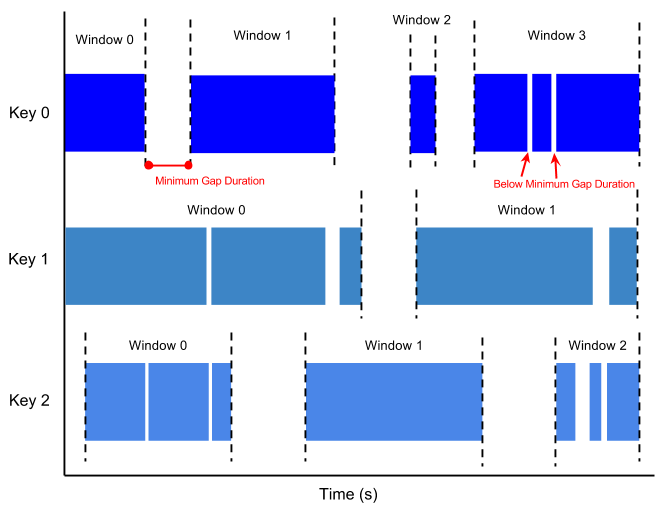## Take aways

Dataflow-style programming can help simplify data processing tasks

Don't be afraid to take a few steps that only re-arrange or re-organize data

Most steps can be run in parallel (and you have no choice to but to write it as such)

Think in terms of computations, not moving data around

Apache Beam provides a consistent and easy way to build dataflow pipelines